Courses

# Test: Conductance - 1

## 20 Questions MCQ Test Physical Chemistry | Test: Conductance - 1

Description
This mock test of Test: Conductance - 1 for Chemistry helps you for every Chemistry entrance exam. This contains 20 Multiple Choice Questions for Chemistry Test: Conductance - 1 (mcq) to study with solutions a complete question bank. The solved questions answers in this Test: Conductance - 1 quiz give you a good mix of easy questions and tough questions. Chemistry students definitely take this Test: Conductance - 1 exercise for a better result in the exam. You can find other Test: Conductance - 1 extra questions, long questions & short questions for Chemistry on EduRev as well by searching above.
QUESTION: 1

### The unit of molar conductivity is:

Solution:
• The molar conductivity of an electrolytic solution is the conductance of the volume of the solution containing a unit mole of electrolyte that is placed between two electrodes of unit area cross-section or at a distance of one-centimeter apart.
• The unit of molar conductivity is S⋅m2⋅mol-1.
QUESTION: 2

### The cell constant of a conductivity cell is defined as: Where l = distance between electrodes and A = area of cross-section of each of the electrodes

Solution:
• The cell constant is a multiplier constant specific to a conductivity sensor.
• The measured current is multiplied by the cell constant to determine the electrical conductivity of the solution.
• The cell constant, known as K, refers to a theoretical electrode consisting of two 1 cm square plates 1 cm apart.
• A cell constant has units of 1/cm (per centimeter), where the number refers to the ratio of the distance between the electrode plates to the surface area of the plate.
QUESTION: 3

### Molar conductivity is defined as: Where k = conductivity, G = conductance, l = distance between two electrodes and Vm = volume of solution containing 1 mol of electrolyte

Solution:
• The molar conductivity of an electrolytic solution is the conductance of the volume of the solution containing a unit mole of electrolyte that is placed between two electrodes of unit area cross-section or at a distance of one-centimeter apart.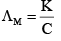Where C = concentration of electrolyte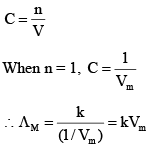QUESTION: 4

For a dilute solution of a strong electrolyte, the variation of molar conductivity with concentration is given by:

Solution:
QUESTION: 5

Which of the following ion is expected to have least value of molar conductivity at infinite dilution in an aqueous solution:

Solution:
•  Na+ has lower molar conductance than the ions that are greater in size than Na such as K+, Cs+ as the molar conductance is inversely proportional to the solvation of ions.
• Smaller the ion, the greater will be the solvation, and hence lower will be the molar conductance.
QUESTION: 6

Which of the following ion is expected to have highest value of molar conductivity at infinite dilution in an aqueous solution:

Solution:

H+ ions will have the maximum molar conductivity amongst the given cations due to Grothus conduction.

QUESTION: 7

The degree of dissociation of a weak electrolyte is given by:

Solution: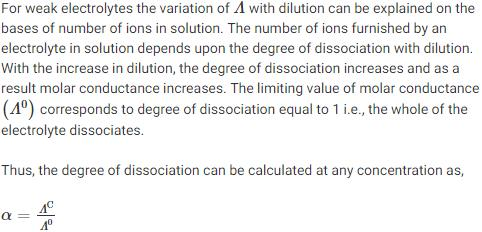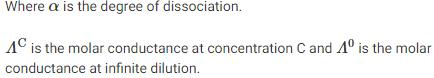QUESTION: 8

Which of the following ion has highest molar conductivity at infinite dilution in an aqueous solution:

Solution:

Exceptionally high values are found for H+ (349.8 S·cm2/mol) and OH (198.6 S·cm2/mol), which are explained by the Grotthuss proton-hopping mechanism for the movement of these ions.

QUESTION: 9

The equilibrium constant of acetic acid in an aqueous solution of concentration C is given by

Solution: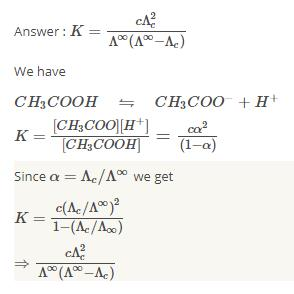QUESTION: 10

Molar conductivity of Fe2(SO4)3 is given by:

Solution:
QUESTION: 11

Equivalent conductivity of Fe2(SO4)3 is related to molar conductivity by the expression:

Solution:
QUESTION: 12

Which of the following expressions is correct:

Solution:

According to kholaraus law,
NH4OH = NH4 + OH
i.e. NH4 + Cl + Na + OH – Na – Cl = NH4Cl + NaOH - NaCl

QUESTION: 13

If x is the specific resistance of the electrolyte solution and y is he molarity of the solution, then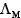is given by:

Solution:

Molar conductivity is defined as the conductivity of an electrolyte solution divided by the molar concentration of electrolyte.

= (k × 1000)/M

= 1/x × 1000/y

= 1000/xy

QUESTION: 14

When a conductance cell was filled with a 0.0025 M solution of K2SO4, its resistance was 326 Ω. If cell constant is 0.2281 cm-1, the specific conductance (Ω-1cm-1) of K2SO4 solution is:

Solution:
QUESTION: 15

Equivalent conductance of 0.1 M HA (weak acid) solution is 10 cm2 equivalent–1. The pH of HA solution is(molar conductivity at infinite dilution is 200).

Solution: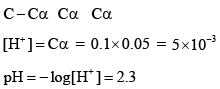QUESTION: 16

For HCl solution at 25ºC, the equivalent conductivity at infinite dilution is 425 Ω-1 cm2 eq-1. The specific conductance of a solution of HCl is 3.825 Ω-1 cm-1. If the apparent degree of dissociat ion is 90%, the normality of solution is

Solution: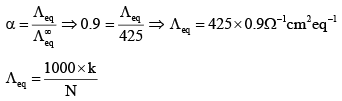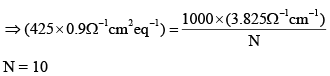QUESTION: 17

The conductance at infinite dilution follow the order

Solution:
• Smaller the ion, the greater will be the solvation, and hence lower will be the molar conductance.
QUESTION: 18

The correct relation which depicts the Debye-Huckel Onsager Theory for strong electrolytes is:

Solution:
• The Debye-Huckel was proposed by Peter Debye and Erich Huckel as a theoretical explanation for departure from ideality in solutions of electrolytes, further modified by Lars Onsager in 1927.
• Onsager was able to derive a theoretical expression to account for the empirical relation known as Kohlrausch's law for the molar conductivity,
Am = Amo - K(c)½

For stronger electrolytes:
Am = Amo - (A + BAmo)(c)1/2ac

QUESTION: 19

The unit of A in Debye-Huckel-Onsager equation for strong electrolytes is:

Solution:
QUESTION: 20

Which of the following solutions will show the minimum value of transference number of Cl–1 ions:

Solution:
• Because tH+ has a maximum value of transference no. as compared to other cations.
• Hence, tCl- will be minimum in 0.1 M HCl solution.